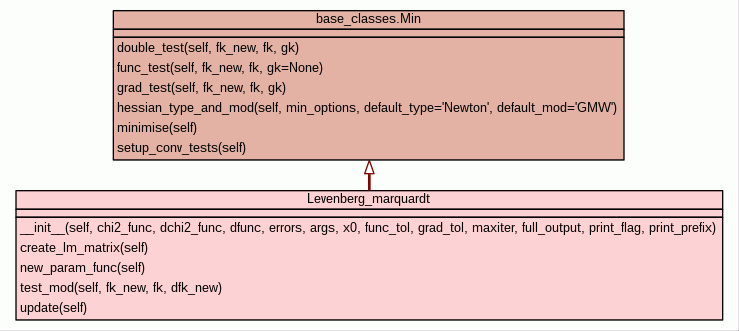[frames] | no frames]

# Class Levenberg_marquardt

source codeInstance Methods

 __init__(self, chi2_func, dchi2_func, dfunc, errors, args, x0, func_tol, grad_tol, maxiter, full_output, print_flag, print_prefix) Class for Levenberg-Marquardt minimisation specific functions. source code

 create_lm_matrix(self) Function to create the Levenberg-Marquardt matrix. source code

 new_param_func(self) Find the new parameter vector self.xk_new. source code

 test_mod(self, fk_new, fk, dfk_new) Modified convergence test. source code

 update(self) Update function source code

Inherited from `base_classes.Min`: `double_test`, `func_test`, `grad_test`, `hessian_type_and_mod`, `minimise`, `setup_conv_tests`

 Method Details

### __init__(self, chi2_func, dchi2_func, dfunc, errors, args, x0, func_tol, grad_tol, maxiter, full_output, print_flag, print_prefix)(Constructor)

source code

Class for Levenberg-Marquardt minimisation specific functions.

Unless you know what you are doing, you should call the function 'levenberg_marquardt' rather than using this class.

Overrides: base_classes.Min.__init__

### create_lm_matrix(self)

source code

Function to create the Levenberg-Marquardt matrix.

The matrix is:

```                  _n_
\   /     1        d y(xi)   d y(xi)                \
LM_matrix_jk =  >  | ---------- . ------- . ------- . (1 + lambda) |
/__ \ sigma_i**2     dj        dk                   /
i=1
```

where j == k is one of the function parameters, and:

```                  _n_
\   /     1        d y(xi)   d y(xi) \
LM_matrix_jk =  >  | ---------- . ------- . ------- |
/__ \ sigma_i**2     dj        dk    /
i=1
```

where j != k are function parameters.

### test_mod(self, fk_new, fk, dfk_new)

source code

Modified convergence test.

This is needed to prevent the Levenberg-Marquardt minimiser from terminating if there is no movement during an iteration due to an uphill step being encountered.

### update(self)

source code

Update function

Update the chi-squared value, chi-squared gradient vector, and derivative function matrix.

Overrides: base_classes.Min.update

 Generated by Epydoc 3.0.1 on Thu Jul 3 13:38:26 2014 http://epydoc.sourceforge.net